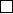5Eigenvalues and eigenvectors

IA Vectors and Matrices5.4 Similar matrices
Definition
(Similar matrices)
.
Two
n ×n
matrices
A
and
B
are similar if there
exists an invertible matrix P such that
B = P
1
AP,
i.e. they represent the same map under different bases. Alternatively, using the
language from IA Groups, we say that they are in the same conjugacy class.
Proposition. Similar matrices have the following properties:
(i) Similar matrices have the same determinant.
(ii) Similar matrices have the same trace.
(iii) Similar matrices have the same characteristic polynomial.
Note that (iii) implies (i) and (ii) since the determinant and trace are the
coefficients of the characteristic polynomial
Proof. They are proven as follows:
(i) det B = det(P
1
AP ) = (det A)(det P )
1
(det P ) = det A
(ii)
tr B = B
ii
= P
1
ij
A
jk
P
ki
= A
jk
P
ki
P
1
ij
= A
jk
(P P
1
)
kj
= A
jk
δ
kj
= A
jj
= tr A
(iii)
p
B
(λ) = det(B λI)
= det(P
1
AP λI)
= det(P
1
AP λP
1
IP )
= det(P
1
(A λI)P )
= det(A λI)
= p
A
(λ)